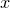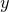## 8.2.2 Error bars

The following pair of plot styles allow datapoints to be plotted with errorbars indicating the uncertainties in either their vertical or horizontal positions:

• yerrorbars

• xerrorbars

Both of these take three columns of input data on 2D plots (or four on 3D plots). The first two (or three) of these represent the-,- (and-) coordinates of the central position of each errorbar, while the last represents the uncertainty in either the- and-coordinate. The plot style errorbars is an alias for yerrorbars. Additionally, the following plot style allows datapoints to be plotted with both horizontal and vertical errorbars:

• xyerrorbars

This plot style takes four (or five) columns of data as input, the first two (or three) of which represent the-,- (and-) coordinates of the central position of each errorbar. The last but one column gives the uncertainty in the-coordinate, and the last column gives the uncertainty in the-coordinate.

Each of the plot styles listed above has a corresponding partner which takes minimum and maximum limits for each errorbar, equivalent to writing, in place of a single symmetric uncertainty:

• xerrorrange – takes four (or five) columns of data.

• yerrorrange – takes four (or five) columns of data.

• xyerrorrange – takes six (or seven) columns of data.

The plot style errorrange is an alias of yerrorrange.

Corresponding plot styles also exist to plot data with errorbars along the-axes of three-dimensional plots: zerrorbars, zerrorrange, xzerrorbars, xzerrorrange, yzerrorbars, yzerrorrange, xyzerrorbars, xyzerrorrange. Though it does not make sense to use these on two-dimensional plots, it is not an error to do so; they expect the same number of columns of input data on both two- and three-dimensional plots.